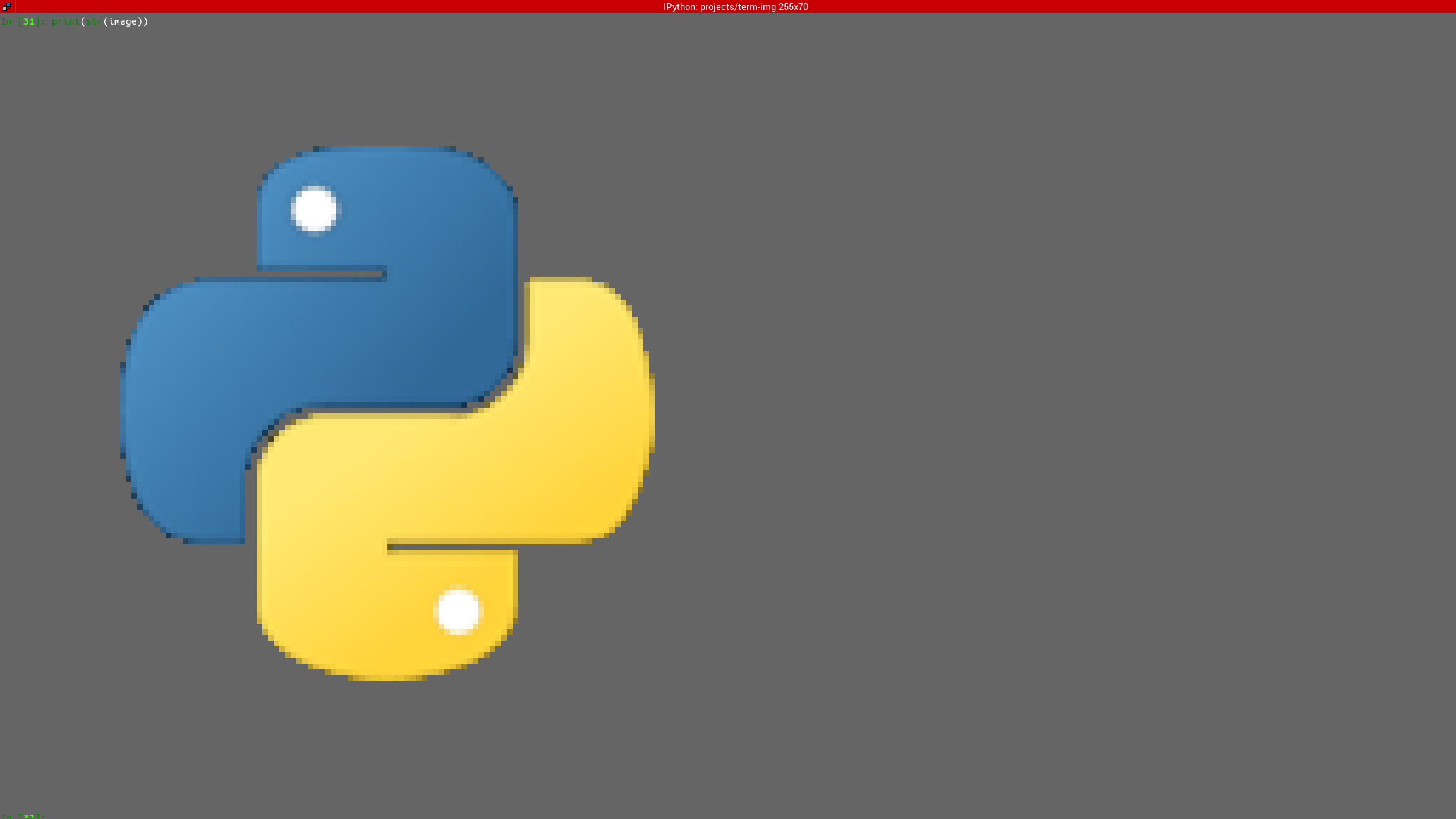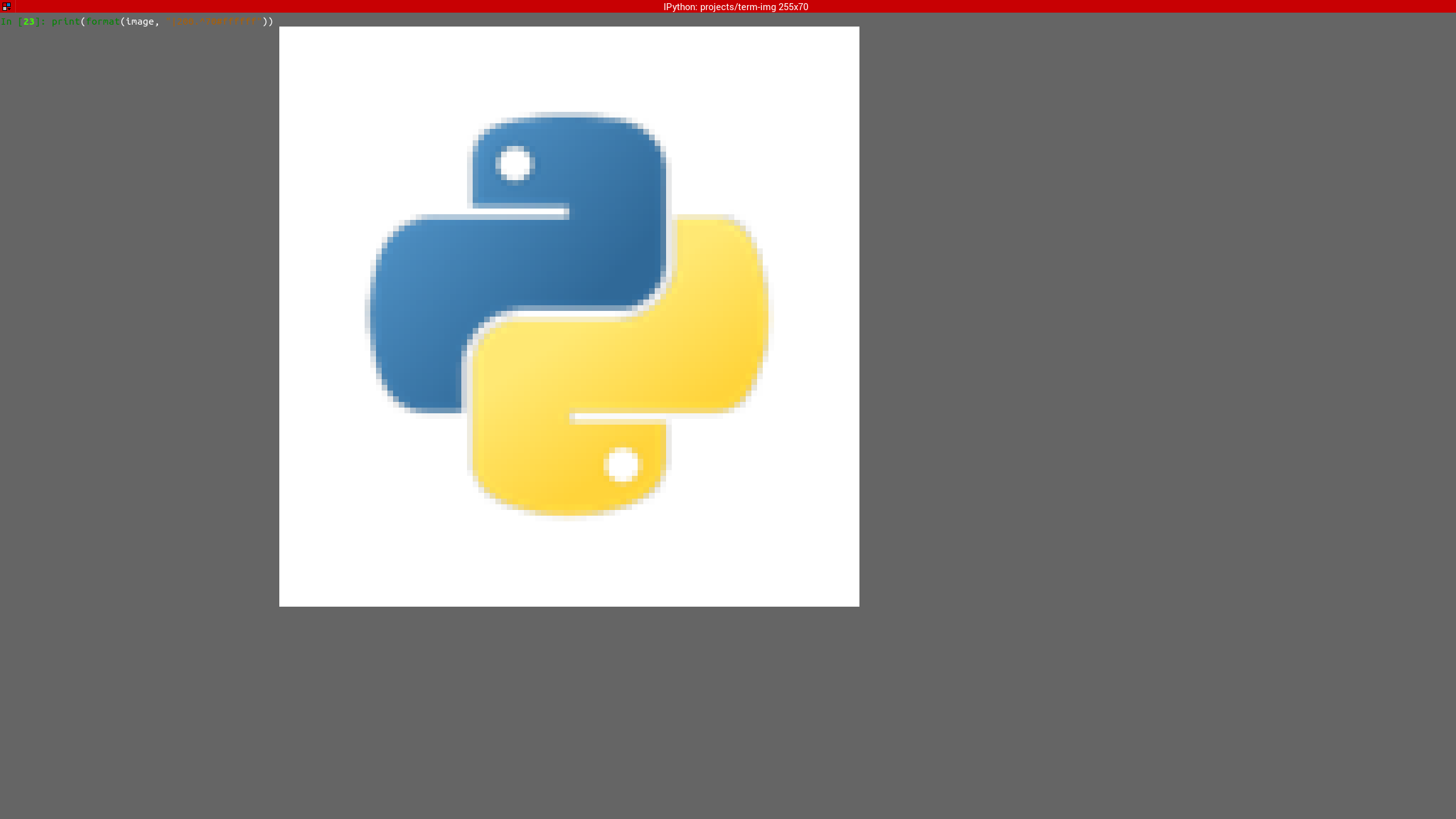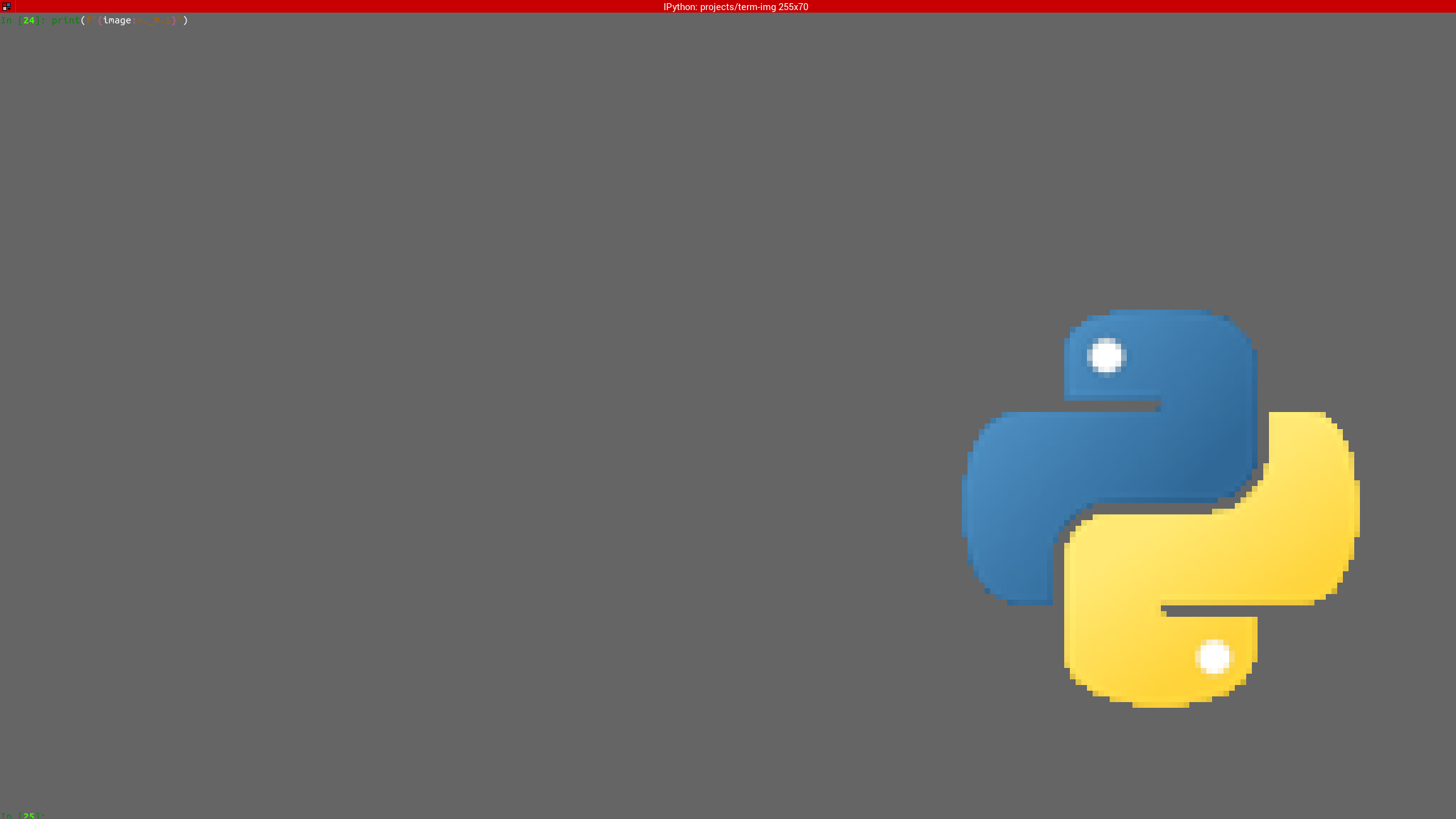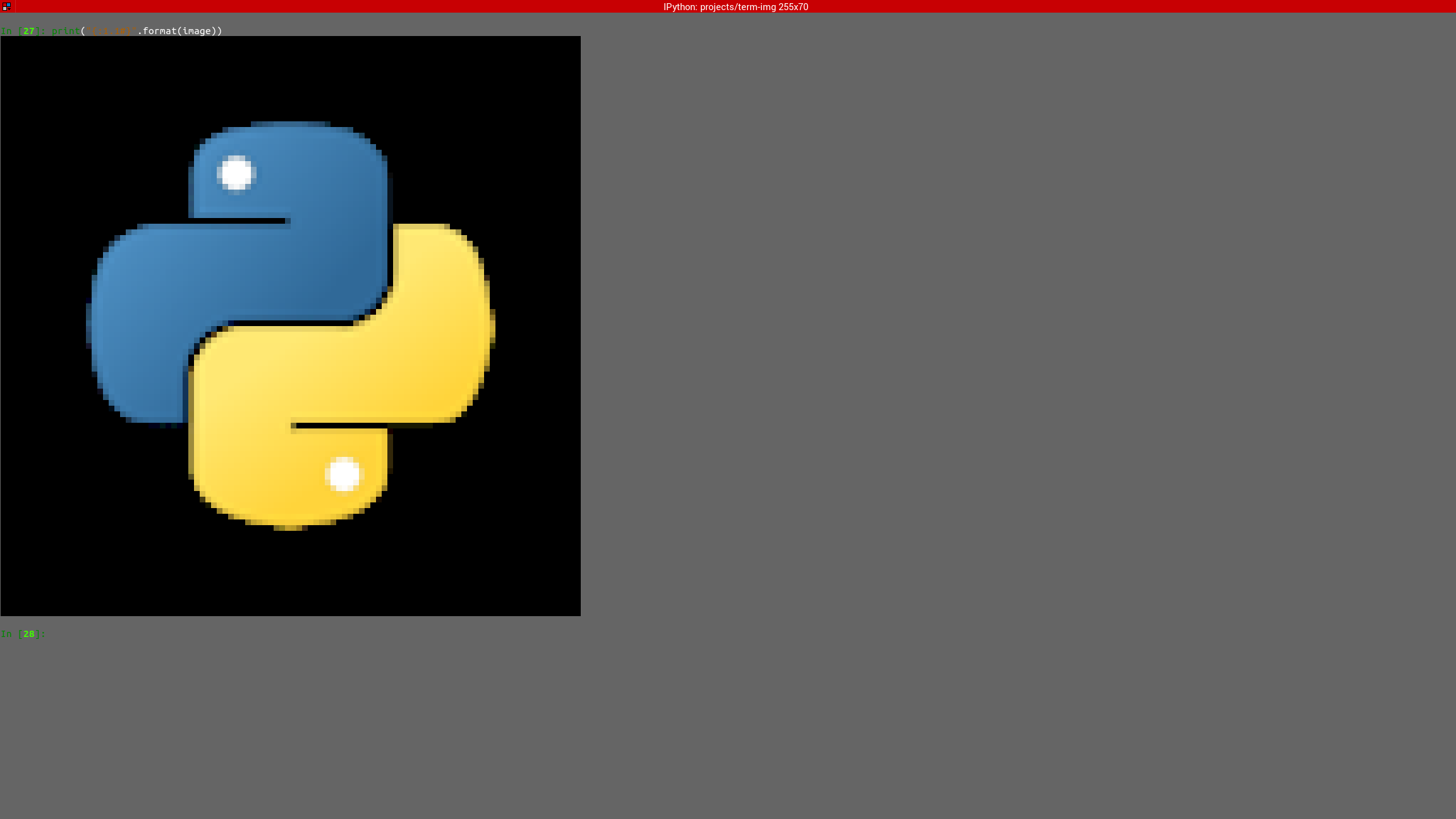# Tutorial

This is a basic introduction to using the library. Please refer to the Reference for detailed description of the features and functionality provided by the library.

For this tutorial we’ll be using the image below:The image has a resolution of 288x288 pixels.

Note

All the samples in this tutorial occured in a terminal window of 255 columns by 70 lines.

## Creating an instance

Image instances can be created using the convinience functions `AutoImage()`, `from_file()` and `from_url()`. These automatically detect the best style supported by the active terminal.

Instances can also be created using the Image Classes directly via their respective constructors or `from_file()` and `from_url()` methods.

If the file is stored on your local filesystem:

```from term_image.image import from_file

image = from_file("path/to/python.png")
```

You can also use a URL if you don’t have the file stored locally:

```from term_image.image import from_url

image = from_url("https://raw.githubusercontent.com/AnonymouX47/term-image/main/docs/source/resources/tutorial/python.png")
```

The library can also be used with PIL image instances:

```from PIL import Image
from term_image.image import AutoImage

img = Image.open("python.png")
image = AutoImage(img)
```

## Rendering an image

Rendering an image is simply the process of converting it (per-frame for animated images) into text (a string).

Hint

To display the rendered image in the following steps, just pass the string as an argument to `print()`.

There are two ways to render an image:

### 1. Unformatted

```str(image)
```

Renders the image without padding/alignment and with transparency enabled

The result should look like:### 2. Formatted

Note

To see the effect of alignment in the steps below, please scale the image down using:

```image.scale = 0.75
```

This simply sets the x-axis and y-axis scales of the image to `0.75`. We’ll see more about this later.

Below are examples of formatted rendering:

```format(image, "|200.^70#ffffff")
```

Renders the image with:

Note

You might have to reduce the padding width (200) and/or height (70) to something that’ll fit into your terminal window, or increase the size of the terminlal window

The result should look like:```f"{image:>._#.5}"
```

Renders the image with:

The result should look like:```"{:1.1#}".format(image)
```

Renders the image with:

• center horizontal alignment (default)

• no horizontal padding, since `1` must be less than or equal to the image width

• middle vertical alignment (default)

• no vertical padding, since `1` is less than or equal to the image height

• transparency disabled (alpha channel is removed)

The result should look like:You should also have a look at the complete Image Format Specification.

## Drawing/Displaying an image to/in the terminal

There are two ways to draw an image to the terminal screen:

1. The `draw()` method

```image.draw()
```

NOTE: `draw()` has various parameters for alignment/padding, transparency, animation control, etc.

2. Using `print()` with an image render output (i.e printing the rendered string)

```print(image)  # Uses str()
```

OR

```print(f"{image:>200.^70#ffffff}")  # Uses format()
```

Note

• For animated images, only the former animates the output, the latter only draws the current frame (see `seek()` and `tell()`).

• Also, the former performs size validation to see if the image will fit into the terminal, while the latter doesn’t.

Important

All the examples above use automatic sizing and default scale.

## Image size

The size of an image is the unscaled dimension with which an image is rendered.
The image size can be retrieved via the `size`, `width` and `height` properties.

The size of an image can be in either of two states:

1. Fixed

In this state, the `size` property is a 2-tuple of integers, the `width` and `height` properties are integers.

2. Dynamic

In this state,

• the size with which the image is rendered is automatically calculated (based on the current terminal size or the image’s original size) whenever the image is to be rendered.

• the `size`, `width` and `height` properties evaluate to a `Size` enum member.

The size of an image can be set when creating an instance by passing an integer or a `Size` enum member to either the width or the height keyword-only parameter.
For whichever axis is given, the other axis is calculated proportionally.

Note

1. The arguments can only be given by keyword.

2. If neither is given, the `FIT` dynamic size applies.

3. All methods of instantiation accept these arguments.

For example:

```>>> from term_image.image import Size, from_file
>>> image = from_file("python.png")  # Dynamic FIT
>>> image.size is Size.FIT
True
>>> image = from_file("python.png", width=60)  # Fixed
>>> image.size
(60, 30)
>>> image.height
30
>>> image = from_file("python.png", height=56)  # Fixed
>>> image.size
(112, 56)
>>> image.width
112
>>> image = from_file("python.png", height=Size.FIT)  # Fixed FIT
>>> image.size
(136, 68)
>>> image = from_file("python.png", width=Size.FIT_TO_WIDTH)  # Fixed FIT_TO_WIDTH
>>> image.size
(255, 128)
>>> image = from_file("python.png", height=Size.ORIGINAL)  # Fixed ORIGINAL
>>> image.size
(288, 144)
```

No size validation is performed i.e the resulting size might not fit into the terminal window

```>>> image = from_file("python.png", height=68)  # Will fit, OK
>>> image.size
(136, 68)
>>> image = from_file("python.png", height=500)  # Will not fit, also OK
>>> image.size
(1000, 500)
```

An exception is raised when both width and height are given.

```>>> image = from_file("python.png", width=100, height=100)
Traceback (most recent call last):
.
.
.
ValueError: Cannot specify both width and height
```

The `width` and `height` properties are used to set the size of an image after instantiation.

```>>> image = from_file("python.png")
>>> image.width = 56
>>> image.size
(56, 28)
>>> image.height
28
>>> image.height = 68
>>> image.size
(136, 68)
>>> image.width
136
>>> image.width = 200  # Even though the terminal can't contain the resulting height, the size is still set
>>> image.size
(200, 100)
>>> image.width = Size.FIT
>>> image.size
(136, 69)
>>> image.height = Size.FIT_TO_WIDTH
>>> image.size
(255, 128)
>>> image.height = Size.ORIGINAL
>>> image.size
(288, 144)
```

Note

An exception is raised if the terminal size is too small to calculate a size.

The `size` property can only be set to a `Size` enum member, which results in a dynamic size.

```>>> image = from_file("python.png")
>>> image.size = Size.FIT
>>> image.size is image.width is image.height is Size.FIT
True
>>> image.size = Size.FIT_TO_WIDTH
>>> image.size is image.width is image.height is Size.FIT_TO_WIDTH
True
>>> image.size = Size.ORIGINAL
>>> image.size is image.width is image.height is Size.ORIGINAL
True
```

Important

1. The currently set cell ratio is also taken into consideration when calculating sizes.

2. There is a default 2-line vertical allowance, to allow for shell prompts or the likes.

Hint

See `set_size()` for extended sizing control.

## Image scale

The scale of an image is the fraction of the size that’ll actually be used to render the image.
A valid scale value is a `float` in the range `0 < x <= 1` i.e greater than zero and less than or equal to one.

The image scale can be retrieved via the properties `scale`, `scale_x` and `scale_y`.

The scale can be set at instantiation by passing a value to the scale keyword-only paramter.

```>>> image = from_file("python.png", scale=(0.75, 0.6))
>>> image.scale
>>> (0.75, 0.6)
```

The drawn image (using `image.draw()`) should look like:If the scale argument is ommited, the default scale `(1.0, 1.0)` is used.

```>>> image = from_file("python.png")
>>> image.scale
>>> (1.0, 1.0)
```

The drawn image (using `image.draw()`) should look like:The properties `scale`, `scale_x` and `scale_y` are used to set the scale of an image after instantiation.
`scale` accepts a tuple of two scale values or a single scale value.
`scale_x` and `scale_y` each accept a single scale value.
```>>> image = from_file("python.png")
>>> image.scale = (.3, .56756)
>>> image.scale
(0.3, 0.56756)
>>> image.scale = .5
>>> image.scale
(0.5, 0.5)
>>> image.scale_x = .75
>>> image.scale
(0.75, 0.5)
>>> image.scale_y = 1.
>>> image.scale
(0.75, 1.0)
```

Finally, to explore more of the library’s features and functionality, check out the Reference section.